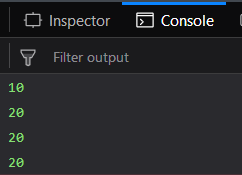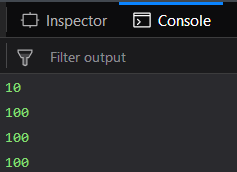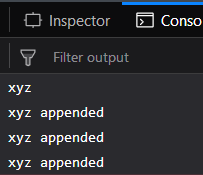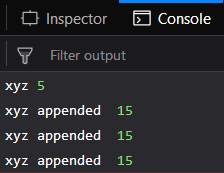GeeksforGeeks App
Open AppBrowser
Continue

# Underscore.js _.once() Function

The Underscore.js is a JavaScript library that provides a lot of useful functions like the map, filter, invoke etc even without using any built-in objects.

The _.once function is used in conditions where we want a particular function to be executed only a single time. Even though we execute or call this function multiple times then also it will have no effect. The original function’s values will only be returned each time it is called.
It is mostly used for the initialize() functions which are used to assign only the initial values to the variables.

Syntax:

`_.once(function)`

Parameters:
It takes only one argument, i.e., the function that needs to be called only a single time.

Return value:
It returns the original call’s value each time the function is iteratively or repeatedly called.

1. Performing addition function with the _.once() function:
The function that is passed to the _.once() function adds 10 to the variable 10 which originally has 10 value. Then the _.once() function is assigned to the another function ‘startFunc()’. Then the first time the ‘startFunc()’ is called the value of ‘a’ is incremented by 10 and becomes 20. So the output of first time calling is 20. Then the next time when ‘startFunc()’ is called the value of ‘a’ is again supposed to be incremented by 10 but this does not happen. This is because the ‘startFunc()’ has a function ‘_.once()’ in its definition which prevents it from being executed more than one times. So, the output of the second and third calls will be the same as first, i.e., 20. On the first line, the value of ‘a’ is getting printed before calling the ‘startFunc()’ hence the output is 10.
Examples:

 `<``html``>`` ` `<``head``>``    ``<``script` `src``=``"https://cdnjs.cloudflare.com/ajax/libs/underscore.js/1.9.1/underscore-min.js"``>``  `````` ` `<``body``>``    ``<``script` `type``=``"text/javascript"``>``        ``var a = 10;`` ` `        ``function add() {``            ``a += 10;``        ``}``        ``var startFunc = _.once(add);``        ``console.log(a);``        ``startFunc();``        ``console.log(a);``        ``startFunc();``        ``console.log(a);``        ``startFunc();``        ``console.log(a);``    `````` ` ``

Output:2. Performing multiplication operation with the _.once() function:
The function that is passed to the _.once() function multiplies by 10 the variable ‘a’ which has value 10 originally. Then the _.once() function is assigned to another function ‘startFunc()’. The first time the ‘startFunc()’ is called the value of ‘a’ is multiplied by 10 and becomes 100. So the output of first time calling is 100. Then the next time when ‘startFunc()’ is called the value of ‘a’ is again supposed to be again multiplied by 10 but this does not happen. This is because the ‘startFunc()’ has a function ‘_.once()’ in its definition which prevents it from being executed more than one times. So, the output of the second and third calls will be the same as first, i.e., 100. On the first line, the value of ‘a’ is getting printed before calling the ‘startFunc()’ hence the output is 10.

 `<``html``>`` ` `<``head``>``    ``<``script` `src``=``"https://cdnjs.cloudflare.com/ajax/libs/underscore.js/1.9.1/underscore-min.js"``>``  `````` ` `<``body``>``    ``<``script` `type``=``"text/javascript"``>``        ``var a = 10;`` ` `        ``function add() {``            ``a *= 10;``        ``}``        ``var startFunc = _.once(add);``        ``console.log(a);``        ``startFunc();``        ``console.log(a);``        ``startFunc();``        ``console.log(a);``        ``startFunc();``        ``console.log(a);``    `````` ` ``

Output:3. Passing strings to the _.once() function:
The function that is passed to the _.once() function appends the original string of the variable ‘a’ with the other string. The _.once() function is assigned to another function ‘startFunc()’. The first time the ‘startFunc()’ is called the value of ‘a’ is appended by ” appended” string and hence becomes “xyz appended”. So the output of first time calling is “xyz appended”. Then the next time when ‘startFunc()’ is called the value of ‘a’ is again supposed to be again appended but this does not happen. This is because the ‘startFunc()’ has a function ‘_.once()’ in its definition which prevents it from being executed more than one times. So, the output of the second and third calls will be the same as first, i.e., “xyz appended”. On the first line, the value of ‘a’ is getting printed before calling the ‘startFunc()’ hence the output is “xyz”.

 `<``html``>`` ` `<``head``>``    ``<``script` `src``=``"https://cdnjs.cloudflare.com/ajax/libs/underscore.js/1.9.1/underscore-min.js"``>``  `````` ` `<``body``>``    ``<``script` `type``=``"text/javascript"``>``        ``var a = 'xyz';`` ` `        ``function add() {``            ``a += " appended ";``        ``}``        ``var startFunc = _.once(add);``        ``console.log(a);``        ``startFunc();``        ``console.log(a);``        ``startFunc();``        ``console.log(a);``        ``startFunc();``        ``console.log(a);``    `````` ` ``

Output:4. Passing both number and string to the _.once() function:
Here we perform _.once() function on a function which both appends the string to a variable ‘a’ whose original value is “xyz” and also adds 10 to a variable ‘b’ whose original value is 5. On the first line the original values of both the variables ‘a’ and ‘b’ will be displayed. After that when we first time call the ‘startFunc()’ the ‘a’ variable is appended by ” appended” string and the ‘b’ variable’s value is incremented by 10. So, ‘a’ becomes “xyz appended” and ‘b’ becomes 15. Now each time the ‘startFunc()’ is used the values of ‘a’ and ‘b’ will remain same as we have use _.once() function in the definition of ‘startFunc()’.

 `<``html``>`` ` `<``head``>``    ``<``script` `src``=``"https://cdnjs.cloudflare.com/ajax/libs/underscore.js/1.9.1/underscore-min.js"``>``  `````` ` `<``body``>``    ``<``script` `type``=``"text/javascript"``>``        ``var a = 'xyz',``            ``b = 5;`` ` `        ``function add() {``            ``a += " appended ";``            ``b += 10;``        ``}``        ``var startFunc = _.once(add);``        ``console.log(a, b);``        ``startFunc();``        ``console.log(a, b);``        ``startFunc();``        ``console.log(a, b);``        ``startFunc();``        ``console.log(a, b);``    `````` ` ``

Output:NOTE:
These commands will not work in Google console or in firefox as for these additional files need to be added which they didn’t have added.
 `<``script` `type``=``"text/javascript"` `src` `=``"https://cdnjs.cloudflare.com/ajax/libs/underscore.js/1.9.1/underscore-min.js"``>```